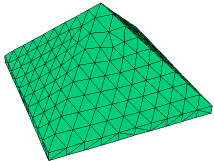﻿ Sample Problems > Usage > 3D_domains > 3d_pyramid

# 3d_pyramid

Navigation:  Sample Problems > Usage > 3D_domains >

# 3d_pyramid{ 3D_PYRAMID.PDE

This problem considers the flow of heat in a pyramid-shaped body.

It demonstrates the use of FlexPDE in 3D problems with non-planar

extrusion surfaces.

Note that FEATURE paths are used to delineate discontinuities in the

extrusion surfaces.

The outer edge is used as a heat source, so it is clipped to form an edge wall.

 }   title '3D Test - Pyramid'   coordinates    cartesian3   select    regrid=off    ngrid=5 { reduce mesh size for example }   variables    u   definitions    k = 0.1    heat = 4   equations    U: div(K*grad(u)) + heat   = 0   extrusion   surface z = 0   surface z = min(1.1- abs(x),1.1-abs(y))boundaries

{ implicit natural(u) = 0 on top and bottom faces }

Region 1

start(-1,-1)

value(u) = 0           { Fixed value on short vertical sides }

line to (1,-1) to (1,1) to (-1,1) to close

{ Features delineate hidden discontinuities in surface slope.

This forces gridding nodes along break lines. }

feature start(-1,-1) line to (1,1)

feature start(-1,1) line to (1,-1)

plots

contour(u) on x=0         as "YZ plane intersects peak"

contour(u) on y=0         as "XZ plane intersects peak"

contour(u) on z=0.1       as "XY plane intersects full outline"

contour(u) on x=0.51       as "YZ plane near midpoint of side slope"

contour(u) on x+y=0.51     as "Oblique plane cuts corner"

contour(u) on z=0.8       as "XY plane near tip"

contour(u) on z=0.8 zoom as "XY plane near tip - zoomed"

end